Next: Planetary Response Up: Terrestrial Ocean Tides Previous: Planetary Rotation

# Total Gravitational Potential

The planet is modeled as a solid body of uniform mass density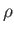whose surface lies at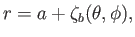(12.45)

where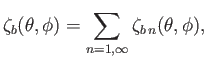(12.46)

and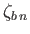is a surface harmonic of degree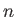. Suppose that the planetary surface is covered by an ocean of uniform mass density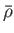whose surface lies at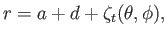(12.47)

where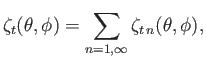(12.48)

and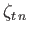is a surface harmonic of degree. Here,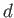is the constant unperturbed depth of the ocean, whereas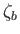and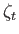are the radial displacements of the ocean's bottom and top surfaces, respectively, generated by planetary rotation and tidal effects. It is assumed that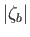,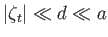.

The net gravitational acceleration in the vicinity of the planet takes the form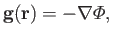(12.49)

where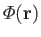is the total gravitational potential. In the limit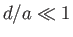, we can write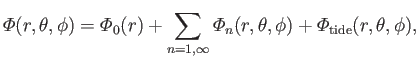(12.50)

where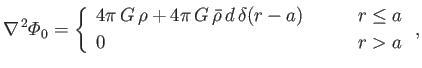(12.51)

and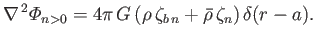(12.52)

Here,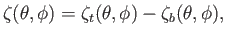(12.53)

is the net change in ocean depth due to planetary rotation and tidal effects, and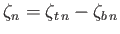. Moreover,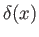is a Dirac delta function (Riley 1974). The boundary conditions are that the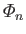be well behaved as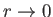, and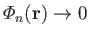(12.54)

as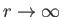. It follows that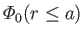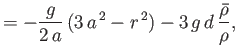(12.55)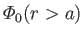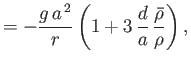(12.56)

and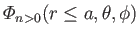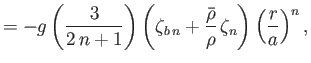(12.57)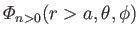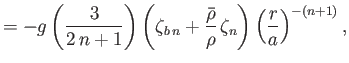(12.58)

where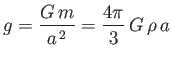(12.59)

is the mean gravitational acceleration at the planet's surface. Note that, inside the planet (i.e.,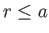),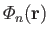is a solid harmonic of degree. We can identify the three terms appearing on the right-hand side of Equation (12.50) as the equilibrium gravitational potential generated by the planet and the ocean, the potential generated by non-spherically-symmetric radial displacements of the planet and ocean surfaces, and the tide generating potential, respectively.Next: Planetary Response Up: Terrestrial Ocean Tides Previous: Planetary Rotation
Richard Fitzpatrick 2016-01-22